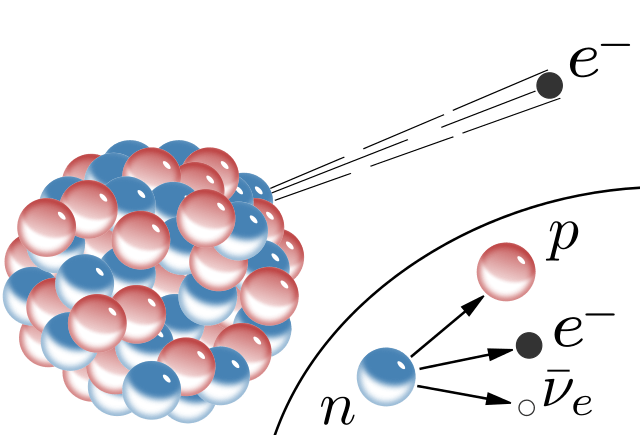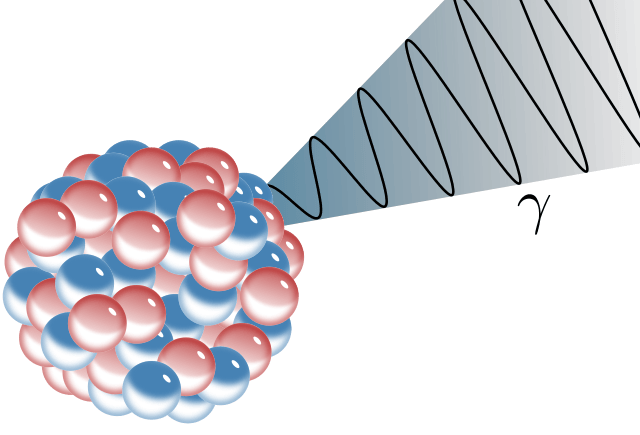# Difference Between Alpha Beta and Gamma Particles

## Main Difference – Alpha vs Beta vs Gamma Particles

Radioactivity is a process of decay of chemical elements with time. This decay occurs through emission of different particles. The emission of particles is also called the emission of radiation. The radiation is emitted from the nucleus of an atom, converting protons or neutrons of the nucleus into different particles. The process of radioactivity takes place in unstable atoms. These unstable atoms undergo radioactivity in order to stabilize themselves. There are three main types of particles that can be emitted as radiation. They are alpha (α) particles, beta (β) particles, and gamma (γ) particles. The main difference between alpha beta and gamma particles is that alpha particles have the least penetration power while beta particles have a moderate penetration power and gamma particles have the highest penetration power.

### Key Areas Covered

1. What are Alpha Particles
– Definition, Properties, Emission Mechanism, Applications
2. What are Beta Particles
– Definition, Properties, Emission Mechanism, Applications
3. What are Gamma Particles
– Definition, Properties, Emission Mechanism, Applications
4. What is the Difference Between Alpha Beta and Gamma Particles
– Comparison of Key Differences## What are Alpha Particles

An alpha particle is a chemical species that is identical to the Helium nucleus and is given the symbol α. Alpha particles are composed of two protons and two neutrons. These alpha particles can be released from the nucleus of a radioactive atom. Alpha particles are emitted in the alpha decay process.

Alpha particle emission occurs in “proton rich” atoms. After the emission of one alpha particle from the nucleus of an atom of a particular element, that nucleus is changed, and it becomes a different chemical element. This is because two protons are removed from the nucleus in the alpha emission, resulting in a reduced atomic number. (The atomic number is the key to identify a chemical element. A change in the atomic number indicates the conversion of one element into another).Figure 1: Alpha Decay

Since there are no electrons in the alpha particle, the alpha particle is a charged particle. The two protons give +2 electrical charge to the alpha particle. The mass of the alpha particle is about 4 amu. Therefore, alpha particles are the biggest particles that are emitted from a nucleus.

However, the penetration power of alpha particles is considerably poor. Even a thin paper can stop alpha particles or alpha radiation. But the ionizing power of alpha particles is very high. Since alpha particles are positively charged, they can easily take electrons from other atoms. This removal of electrons from other atoms causes those atoms to get ionized. Since these alpha particles are charged particles, they are easily attracted by electric fields and magnetic fields.

## What are Beta Particles

A beta particle is a high-speed electron or a positron. The symbol for beta particle is β. These beta particles are released from “neutron rich” unstable atoms. These atoms get a stable state by removing the neutrons and converting them into electrons or positrons. The removal of a beta particle changes the chemical element. A neutron is converted into a proton and a beta particle. Therefore, the atomic number is increased by 1. Then it becomes a different chemical element.

A beta particle is not an electron from the outer electron shells. These are generated in the nucleus. An electron is negatively charged and a positron is positively charged. But positrons are identical to electrons. Therefore, the beta decay occurs in two ways as β+ emission and β- emission. β+ emission involves the emission of positrons. β- emission involves the emission of electrons.Figure 2: β- Emission

Beta particles are able to penetrate air and paper, but can be stopped by a thin metal (such as aluminum) sheet. It can ionize the matter it meets. Since they are negatively (or positively if it is a positron) charged particles, they can repel electrons in other atoms. This results in the ionization of matter.

Since these are charged particles, beta particles are attracted by electrical fields and magnetic fields. The speed of a beta particle is about 90% of the speed of light. Beta particles are able to penetrate human skin.

## What are Gamma Particles

Gamma particles are photons that carry energy in the form of electromagnetic waves. Therefore, gamma radiation is not composed of actual particles. Photons are hypothetical particles. Gamma radiation is emitted form unstable atoms. These atoms get stabilized by removing the energy as photons in order to obtain a lower energy state.

The gamma radiation is high frequency and low wavelength electromagnetic radiation. Photons or the gamma particles are not electrically charged and are not affected by magnetic fields or electrical fields. Gamma particles have no mass. Therefore, the atomic mass of the radioactive atom is not reduced or increased by gamma particle emission. Therefore, the chemical element is not changed.

The penetrating power of gamma particles is very high. Even very small radiation can penetrate through air, papers and even thin metal sheets.Figure 3: Gamma Decay

Gamma particles are removed along with alpha or beta particles. Alpha or beta decay may change the chemical element but cannot change the energy state of the element. Therefore, if the element is still in a higher energy state, then the gamma particle emission occurs in order to obtain a lower energy level.

## Difference Between Alpha Beta and Gamma Particles

### Definition

Alpha Particles: An alpha particle is a chemical species that is identical to the Helium nucleus.

Beta Particles: A beta particle is a high speed electron or a positron.

Gamma Particles: A gamma particle is a photon that carries energy in the form of electromagnetic waves.

### Mass

Alpha Particles: The mass of an alpha particle is about 4 amu.

Beta Particles: The mass of a beta particle is about 5.49 x 10-4 amu.

Gamma Particles: Gamma particles has no mass.

### Electrical Charge

Alpha Particles: Alpha particles are positively charged particles.

Beta Particles: Beta particles are either positively or negatively charged particles.

Gamma Particles: Gamma particles are not charged particles.

### Effect on the Atomic Number

Alpha Particles: The atomic number of element is reduced by 2 units when an alpha particle is released.

Beta Particles: The atomic number of element is increased by 1 unit when a beta particle is released.

Gamma Particles: The atomic number is not affected by gamma particle emission.

### Change in the Chemical Element

Alpha Particles: Alpha particle emission cause the chemical element to be changed.

Beta Particles: Beta particle emission cause the chemical element to be changed.

Gamma Particles: Gamma particle emission does not cause the chemical element to be changed.

### Penetration Power

Alpha Particles: Alpha particles have the least penetration power.

Beta Particles: Beta particles have a moderate penetration power.

Gamma Particles: Gamma particles have the highest penetration power.

### Ionizing Power

Alpha Particles: Alpha particles can ionize many other atoms.

Beta Particles: Beta particles can ionize other atoms, but is not good as alpha particles.

Gamma Particles: Gamma particles have the least ability to ionize other matter.

### Speed

Alpha Particles: The speed of alpha particles is about tenth of the speed of light.

Beta Particles: The speed of beta particle is about 90% of the speed of light.

Gamma Particles: The speed of gamma particles is equal to the speed of light.

### Electrical and Magnetic Fields

Alpha Particles: Alpha particles are attracted by electrical and magnetic fields.

Beta Particles: Beta particles are attracted by electrical and magnetic fields.

Gamma Particles: Gamma particles are not attracted by electrical and magnetic fields.

### Conclusion

Alpha, beta and gamma particles are emitted from unstable nuclei. A nucleus emit these different particles in order to become stable. Although alpha and beta rays are composed of particles, gamma rays are not composed of actual particles. However, in order to understand the behavior of gamma rays and to compare them with alpha and beta particles, a hypothetical particle called photon is introduced. These photons are energy packets that transport energy from one place to another as a gamma ray. Therefore, they are called gamma particles. The main difference between alpha beta and gamma particles is their penetrating power.

##### References:

1. “GCSE Bitesize: Types of radiation.” BBC, Available here. Accessed 4 Sept. 2017.
2. “Gamma Radiation.” NDT Resource center, Available here. Accessed 4 Sept. 2017.
3. “Types of Radiation: Gamma, Alpha, Neutron, Beta & X-Ray Radiation Basics.” Mirion, Available here. Accessed 4 Sept. 2017.

##### Image Courtesy:

1. “Alpha Decay” Von Inductiveload – Eigenes Werk (Gemeinfrei) via Commons Wikimedia
2. “Beta-minus Decay” Von Inductiveload – Eigenes Werk (Gemeinfrei) via Commons Wikimedia
3. “Gamma Decay” By Inductiveload – self-made (Public Domain) via Commons Wikimedia